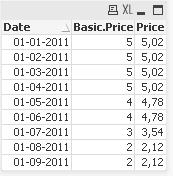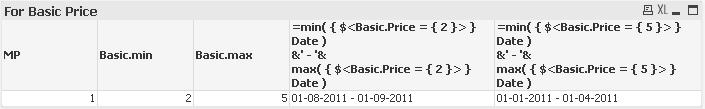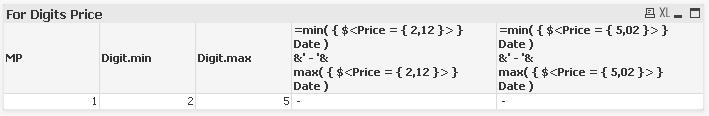QlikView App Development

Discussion Board for collaboration related to QlikView App Development.

Announcements
HighlightedNot applicable

Set analysis problem with value formats

I have an urgent  problem with set anlayis. I have to monitor price changes and need to know for how long the lowes/highest price was valid. I build some test case and it works fine for numbers without digits but not for numbers with digits nor for my real data.

Example:With the Basic price the following works fine (in words: search the min.date for the min.Price):

=min( {\$<(Basic.Price = { \$(=min(Basic.Price)) }> } Date )&' - '&max( { \$<Basic.Price = { \$(=min(Basic.Price)) }> } Date )However, with numbers with digits it does not work:The function min(Price) gives back the correct value of 2,12 but this as input for the selection in the SET seems to be a problem....

I attached the example QV-file.

Any ideas ?

Christian

Tags (3)
1 Solution

Accepted SolutionsMVP

Re: Set analysis problem with value formats

Hi,

I think the returned comma of min(Price) is problematic and the set element expression should use '' like

=min( { \$<Price = {'\$(=min(Price))'}> } Date )

&' - '&

max( { \$<Price = {'\$(=min(Price))'}> } Date )

(Note that I guarded the dollar sign expression with ' '. That way it works for me.

Stefan

5 RepliesMVP

Re: Set analysis problem with value formats

Hi,

I think the returned comma of min(Price) is problematic and the set element expression should use '' like

=min( { \$<Price = {'\$(=min(Price))'}> } Date )

&' - '&

max( { \$<Price = {'\$(=min(Price))'}> } Date )

(Note that I guarded the dollar sign expression with ' '. That way it works for me.

Stefan

Contributor III

Re: Set analysis problem with value formats

Hi Christian,

Please find the attached solution for the issue.

=min( { \$<Price = { '\$(=min(Price))' }> } Date )

&' - '&

max( { \$<Price = { '\$(=min(Price))' }> } Date )

Let me know if this solves your issue.

Regards,

HNot applicable

Re: Set analysis problem with value formats

Thank you both, this works for my example. No I realize the next issue:

When I add a second product (field MP has now >1 value) the set-analysis ignores this dimension in the chart-table. As a result I find the only the date when the minPrice is the absolute min, not the min for the product.How to take into account the dimension ?

(Attached an updated example)MVP

Re: Set analysis problem with value formats

Hi,

I believe what you want to achieve is quite hard using set analysis, if possible at all.

But using advanced aggregation, it's possible.

Please have a look at my attached sample.

Regards,

StefanNot applicable

Re: Set analysis problem with value formats

Hi Stefan,

thank you very much !!

This completely solves the problem (even if the aggr-function took a bit longer to understand...)

Let's hope, that QV implements a set analysis also interpreting the chart dimension as per line selection to be added to the set-analysis selection in a future version...

Again, thank you

Christian